# 美团面试题：String s = new String("111")会创建几个对象？

今日推荐编程大佬是否能记住代码，不用百度就能啪啪啪敲出来么？在 IDEA 中的各种调试技巧，轻松定位 Bug（超级全面）



## String不可变吗？public class App {
public static void main(String[] args) {
String a = "111";
a = "222";
System.out.println(a);
}
}


222

Stirng a="111"；


User user = new User()


String 为什么是不可变的？public final class String implements java.io.Serializable, Comparable<String>, CharSequence {
private final char value[];
public String() {
this.value = "".value;
}
public String(String original) {
this.value = original.value;
this.hash = original.hash;
}
public String(char value[]) {
this.value = Arrays.copyOf(value, value.length);
}
}


String 类是final修饰

String存储内容使用的是char数组

char数组是final修饰

## 这里就得复习一下，final有啥用？

/**
* Description: final修饰变量
* @author : 田维常
* 欢迎关注：java后端技术全栈
*/
public class FinalDemo {
private final String name;

public FinalDemo(String name) {
this.name = name;
}

public FinalDemo() {
}
}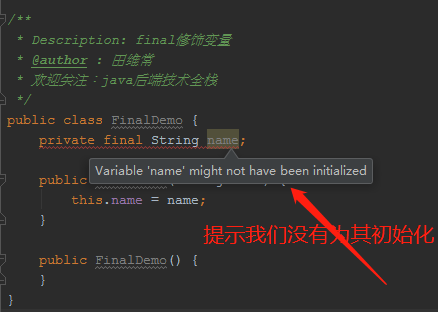/**
* Description:
*
* @author : 田维常
* @date : 2020/11/3
* 欢迎关注公众号：java后端技术全栈
*/
public class StringDemo {
public static void main(String[] args) {
String name = "老田";
name.concat("!");
System.out.println(name);
System.out.println(name.concat("!"));
}
}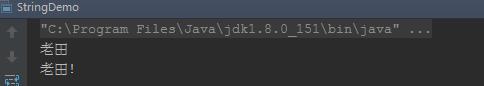public String concat(String str) {
int otherLen = str.length();
if (otherLen == 0) {
//啥都没有，就直接把当前字符串给你
return this;
}
int len = value.length;
char buf[] = Arrays.copyOf(value, len + otherLen);
str.getChars(buf, len);
//看到了吗？返回的居然是新的String对象
return new String(buf, true);
}
void getChars(char dst[], int dstBegin) {
System.arraycopy(value, 0, dst, dstBegin, value.length);
}
public String replace(char oldChar, char newChar) {
//如果两个是一样的，那就必要替换了，所以返回this
if (oldChar != newChar) {
int len = value.length;
int i = -1;
//把当前的char数组复制给val，然后下面基于val来操作
char[] val = value;

while (++i < len) {
if (val[i] == oldChar) {
break;
}
}
if (i < len) {
//创建一个新的char数组
char buf[] = new char[len];
for (int j = 0; j < i; j++) {
buf[j] = val[j];
}
while (i < len) {
char c = val[i];
buf[i] = (c == oldChar) ? newChar : c;
i++;
}
//创建一个新的String对象
return new String(buf, true);
}
}
return this;
}

public String substring(int beginIndex, int endIndex) {
if (beginIndex < 0) {
throw new StringIndexOutOfBoundsException(beginIndex);
}
if (endIndex > value.length) {
throw new StringIndexOutOfBoundsException(endIndex);
}
int subLen = endIndex - beginIndex;
if (subLen < 0) {
throw new StringIndexOutOfBoundsException(subLen);
}
//正常返回的都是新new出来的String对象
return ((beginIndex == 0) && (endIndex == value.length)) ? this
: new String(value, beginIndex, subLen);
}

public String trim() {
int len = value.length;
int st = 0;
char[] val = value;    /* avoid getfield opcode */

while ((st < len) && (val[st] <= ' ')) {
st++;
}
while ((st < len) && (val[len - 1] <= ' ')) {
len--;
}
//如果是该字符串中包含了空格，调用substring方法，否则就是啥都没干原本返回
//就是如果字符串里有空格，那么还是新生一个String对象返回
return ((st > 0) || (len < value.length)) ? substring(st, len) : this;
}


String对象一旦被创建就是固定不变的了，对String对象的任何改变都不影响到原对象，相关的任何变化性的操作都会生成新的对象。

String对象每次有变化性操作的时候，都会从新new一个String对象（这里指的是有变化的情况）。

//String a = "111";相当于
char data [] ={'1','1','1'};
Stirng a = new String(data);
//a = "222";
char data [] ={'2','2','2'};
a = new String(data);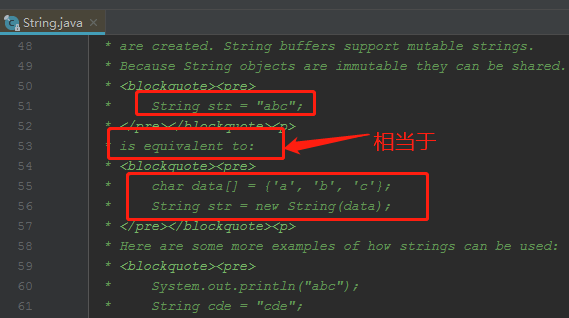public class App {
public static void main(String[] args) {
String a = "111";
String a1 = "111";

String b = new String("111");

//对象地址是同一个
System.out.println(a==a1);
//对象内容是一样的
System.out.println(a.equals(a1));
//对象地址不一样
System.out.println(a==b);
//对象内容是一样的
System.out.println(a.equals(b));
}
}


true
true
false
true


a和a1放在栈上，存放着对象的引用地址。

new的对象是在堆中。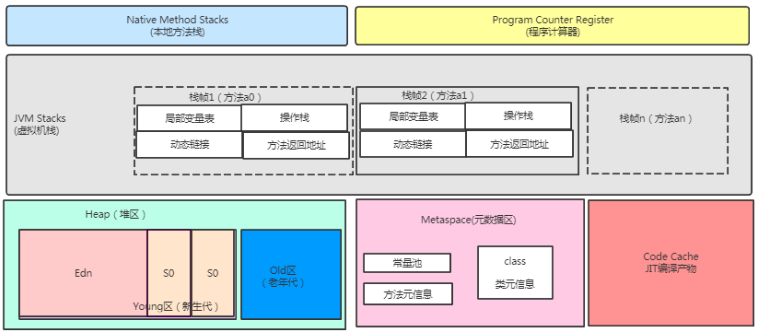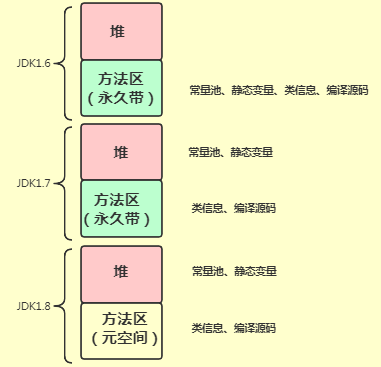推荐文章
更多项目源码


07-1518
06-1484©️2020 CSDN 皮肤主题: Age of Ai 设计师:meimeiellie点击重新获取扫码支付1.余额是钱包充值的虚拟货币，按照1:1的比例进行支付金额的抵扣。
2.余额无法直接购买下载，可以购买VIP、C币套餐、付费专栏及课程。余额充值